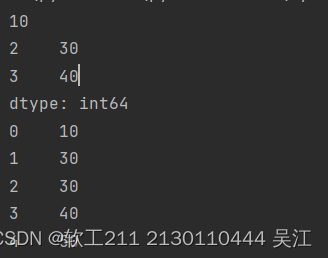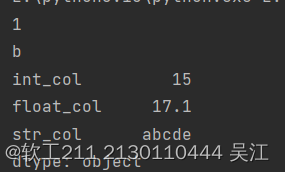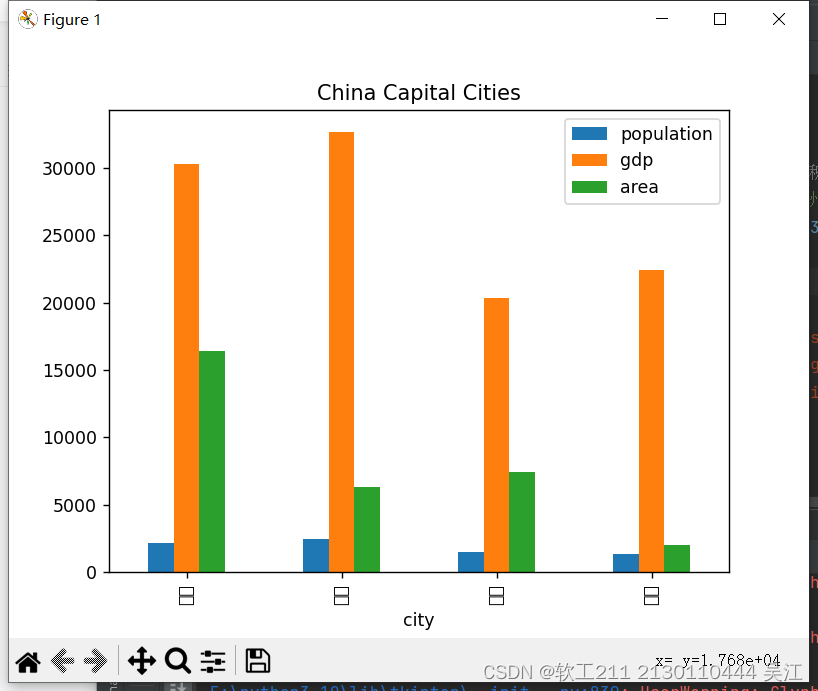# 2023（春）Python程序设计作业5：Pandas基础技能及综合应用

### 实验一：Series对象的应用

#### 实验要求：

• 定义一个Series对象，包含5个整数数据；
• 访问、修改Series对象中的数据；
• 打印Series对象；
• 对Series对象进行计算，如求和、求平均值等。
• 代码：
• import pandas as pd

# 定义一个Series对象
s = pd.Series([10, 20, 30, 40, 50])

# 访问Series对象中的数据
print(s)  # 输出第一个元素
print(s[2:4])  # 输出第3个到第4个元素

# 修改Series对象中的数据
s = 30

# 打印Series对象
print(s)

# 对Series对象进行计算
print(s.sum())  # 求和
print(s.mean())  # 求平均值


结果：

•### 实验二：DataFrame对象的应用

#### 实验要求：

• 定义一个DataFrame对象，包含3个列，每列分别为整数、浮点数和字符串类型；
• 访问、修改DataFrame对象中的数据；
• 对DataFrame对象进行计算，如求和、求平均值等。
• 代码：
• import pandas as pd

# 定义一个DataFrame对象
data = {'int_col': [1, 2, 3, 4, 5], 'float_col': [1.2, 2.3, 3.4, 4.5, 5.6], 'str_col': ['a', 'b', 'c', 'd', 'e']}
df = pd.DataFrame(data)

# 访问DataFrame对象中的数据
print(df['int_col'])  # 输出第一行第一列的数据
print(df.loc[1, 'str_col'])  # 输出第二行第三列的数据

# 修改DataFrame对象中的数据
df.loc[2, 'float_col'] = 3.5

# 对DataFrame对象进行计算
print(df.sum())  # 求和
print(df.mean())  # 求平均值

结果：### 实验三：综合实例

#### 实验要求：

• 定义一个包含省会城市、人口、GDP、城市面积的DataFrame对象；
• 计算各种排名，如人口最多的城市、GDP最高的城市等；
• 使用Pandas绘图，可视化上述实验结果。
• 代码：
• import pandas as pd
import matplotlib.pyplot as plt

# 定义一个包含省会城市、人口、GDP、城市面积的DataFrame对象
data = {'city': ['北京', '上海', '广州', '深圳'], 'population': [2171, 2424, 1500, 1303],
'gdp': [30320, 32679, 20353, 22458], 'area': [16410, 6340, 7434, 1996]}
df = pd.DataFrame(data)

# 计算各种排名
pop_rank = df['population'].rank(ascending=False)
gdp_rank = df['gdp'].rank(ascending=False)
area_rank = df['area'].rank(ascending=False)

# 将排名添加到DataFrame对象中
df['pop_rank'] = pop_rank
df['gdp_rank'] = gdp_rank
df['area_rank'] = area_rank

# 使用Pandas绘图，可视化实验结果
df.plot(kind='bar', x='city', y=['population', 'gdp', 'area'], title='China Capital Cities')
plt.show()

结果：11-25391
11-27329
11-231510
11-26197
11-23496
11-22576
11-28580
11-23220
11-23447
11-23690
11-24173
11-22109
11-24240
11-25539

### “相关推荐”对你有帮助么？

•非常没帮助
•没帮助
•一般
•有帮助
•非常有帮助被折叠的  条评论 为什么被折叠?到【灌水乐园】发言¥1 ¥2 ¥4 ¥6 ¥10 ¥20获取中扫码支付点击重新获取扫码支付1.余额是钱包充值的虚拟货币，按照1:1的比例进行支付金额的抵扣。
2.余额无法直接购买下载，可以购买VIP、付费专栏及课程。余额充值